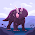## Micaela's rate problem, unit rate problem

Rate to Unit Rate

If there's 6 girls per 2 boys how many girls If there's only one boy?

Rate to Unit Rate

If there's 2 cookies per glass of milk how many glasses of milk If there's 4 cookies?

Unit Rate to Rate

If there's 2 paper per pencil how many paper if there's 10 pencil?

Unit Rate to Rate

If there's 20 apples per milk how many glasses of milk?
6:38 AM

#### 2 comments :

1.the answer for the first question is 3 because 6/3=2 and then 3/3=1 X=3

If there's 8 girls per 4 boys how many girls If there's only 2 boy?

2.Here's the answer for question no.2
~If there's 4 cookies there would be 2 milk/milks. So its 4 cookies per 2 glasses of milk.

Here's a Question:
If there's 5 cookies per glass of milk how many glasses of milk If there's 15 cookies?